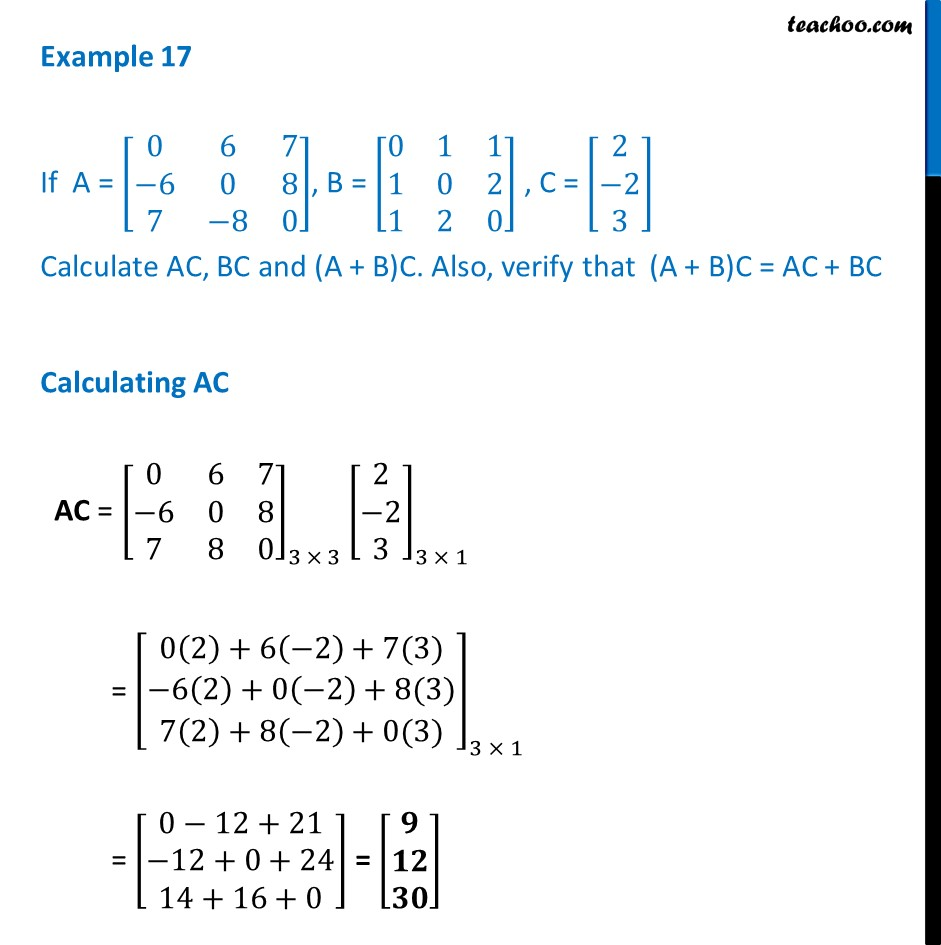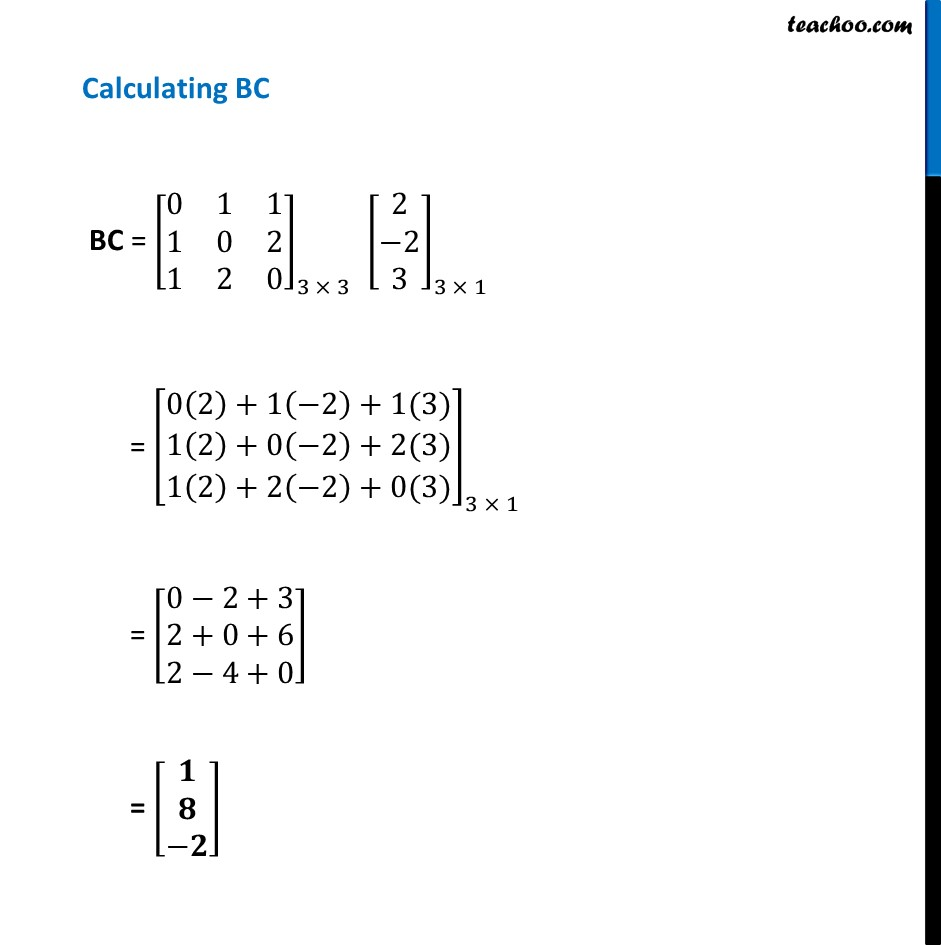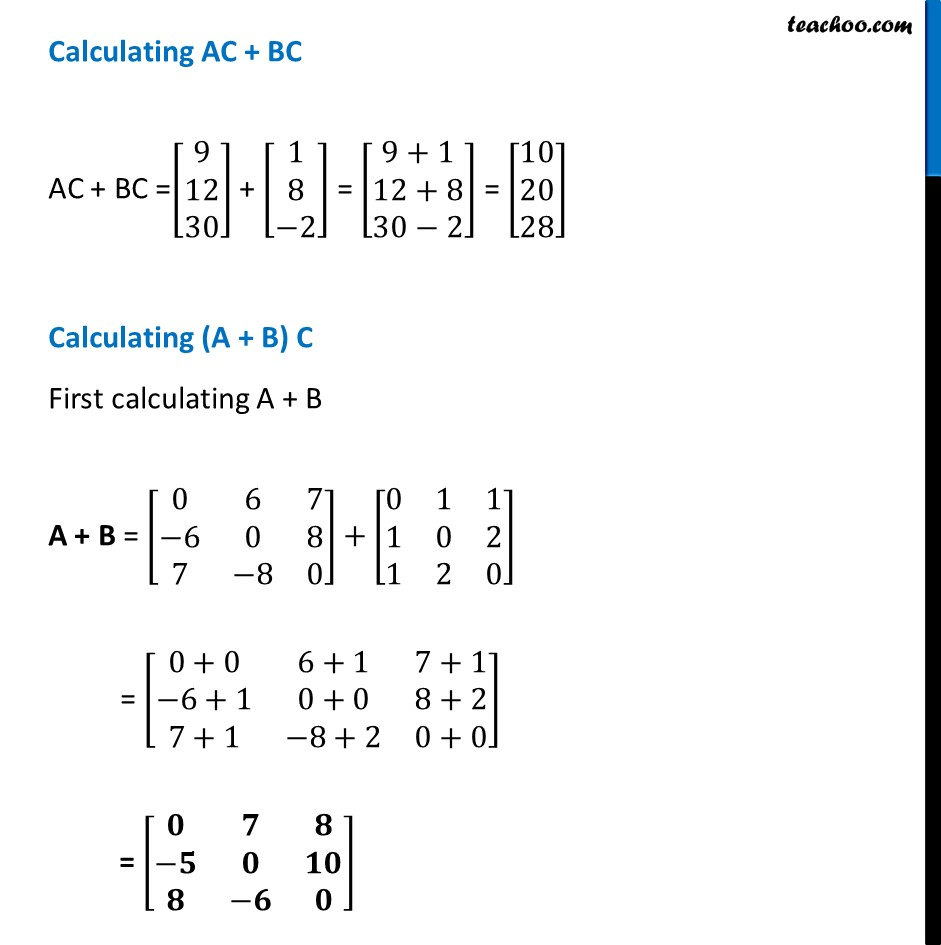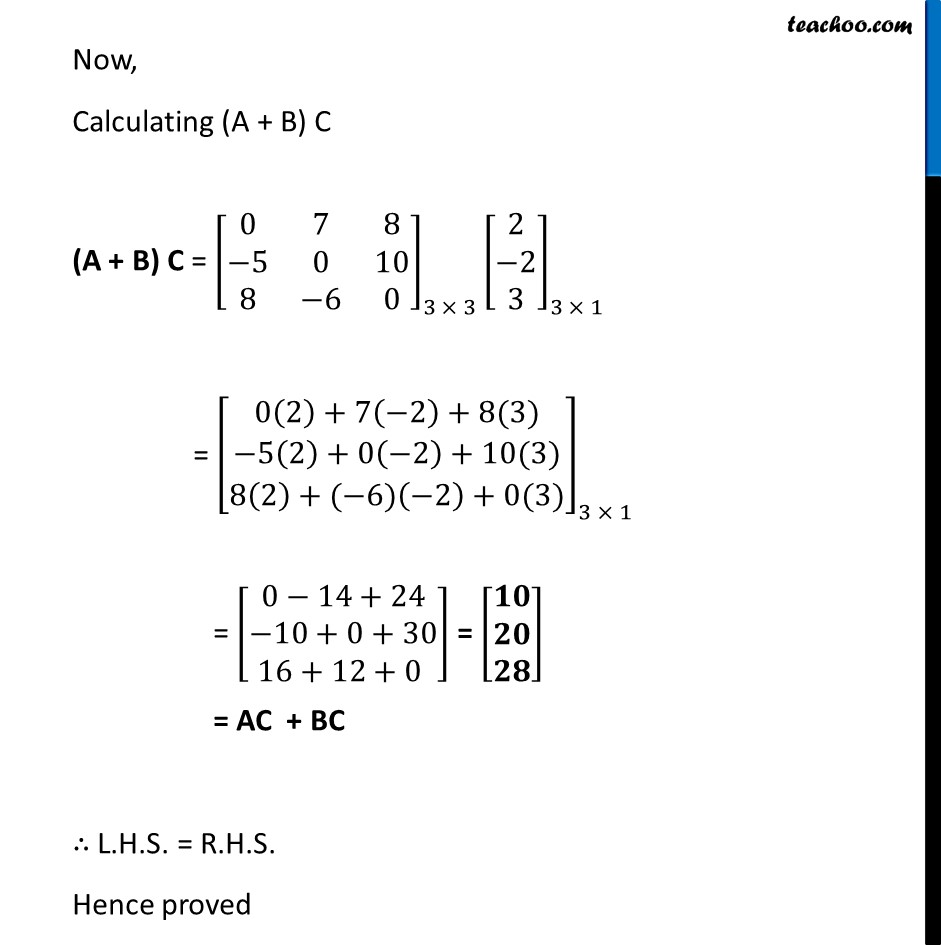Examples

Chapter 3 Class 12 Matrices
Serial order wiseLearn in your speed, with individual attention - Teachoo Maths 1-on-1 Class

### Transcript

Example 17 If A = [■8(0&6&7@−6&0&[email protected]&−8&0)], B = [■8(0&1&[email protected]&0&[email protected]&2&0)] , C = [█(2@−[email protected])] Calculate AC, BC and (A + B)C. Also, verify that (A + B)C = AC + BC Calculating AC AC = [■8(0&6&7@−6&0&[email protected]&8&0)]_(3 × 3) [█(2@−[email protected])]_(3 × 1) = [█(0(2)+6(−2)+7(3)@−6(2)+0(−2)+8(3)@7(2)+8(−2)+0(3))]_(3 × 1) = [█(0−12+21@−[email protected]+16+0)] = [█(𝟗@𝟏𝟐@𝟑𝟎)] Calculating BC BC = [■8(0&1&[email protected]&0&[email protected]&2&0)]_(3 × 3) [█(2@−[email protected])]_(3 × 1) = [█(0(2)+1(−2)+1(3)@1(2)+0(−2)+2(3)@1(2)+2(−2)+0(3))]_(3 × 1) = [█(0−[email protected][email protected]−4+0)] = [█(𝟏@𝟖@−𝟐)] Calculating AC + BC AC + BC =[█([email protected]@30)] + [█([email protected]@−2)] = [█([email protected][email protected]−2)] = [█([email protected]@28)] Calculating (A + B) C First calculating A + B A + B = [■8(0&6&7@−6&0&[email protected]&−8&0)] +[■8(0&1&[email protected]&0&[email protected]&2&0)] = [■8(0+0&6+1&7+1@−6+1&0+0&[email protected]+1&−8+2&0+0)] = [■8(𝟎&𝟕&𝟖@−𝟓&𝟎&𝟏𝟎@𝟖&−𝟔&𝟎)] Now, Calculating (A + B) C (A + B) C = [■8(0&7&8@−5&0&[email protected]&−6&0)]_(3 × 3) [█(2@−[email protected])]_(3 × 1) = [█(0(2)+7(−2)+8(3)@−5(2)+0(−2)+10(3)@8(2)+(−6)(−2)+0(3))]_(3 × 1) = [█(0−14+24@−[email protected]+12+0 )] = [█(𝟏𝟎@𝟐𝟎@𝟐𝟖)] = AC + BC ∴ L.H.S. = R.H.S. Hence proved